Prealgebra 2e

# 1.5Divide Whole Numbers

Prealgebra 2e1.5 Divide Whole Numbers

### Learning Objectives

By the end of this section, you will be able to:

• Use division notation
• Model division of whole numbers
• Divide whole numbers
• Translate word phrases to math notation
• Divide whole numbers in applications

### Be Prepared 1.7

Before you get started, take this readiness quiz.

Multiply: $27·3.27·3.$
If you missed this problem, review Example 1.44.

### Be Prepared 1.8

Subtract: $43−26.43−26.$
If you missed this problem, review Example 1.32

### Be Prepared 1.9

Multiply: $62(87).62(87).$
If you missed this problem, review Example 1.45.

### Use Division Notation

So far we have explored addition, subtraction, and multiplication. Now let’s consider division. Suppose you have the $1212$ cookies in Figure 1.13 and want to package them in bags with $44$ cookies in each bag. How many bags would we need?

Figure 1.13

You might put $44$ cookies in first bag, $44$ in the second bag, and so on until you run out of cookies. Doing it this way, you would fill $33$ bags.In other words, starting with the $1212$ cookies, you would take away, or subtract, $44$ cookies at a time. Division is a way to represent repeated subtraction just as multiplication represents repeated addition.

Instead of subtracting $44$ repeatedly, we can write

$12÷412÷4$

We read this as twelve divided by four and the result is the quotient of $1212$ and $4.4.$ The quotient is $33$ because we can subtract $44$ from $1212$ exactly $33$ times. We call the number being divided the dividend and the number dividing it the divisor. In this case, the dividend is $1212$ and the divisor is $4.4.$

In the past you may have used the notation $412412$, but this division also can be written as $12÷4,12/4,124.12÷4,12/4,124.$ In each case the $1212$ is the dividend and the $44$ is the divisor.

### Operation Symbols for Division

To represent and describe division, we can use symbols and words.

Operation Notation Expression Read as Result
$DivisionDivision$ $÷÷$
$abab$
$baba$
$a/ba/b$
$12÷412÷4$
$124124$
$412412$
$12/412/4$
$Twelve divided by fourTwelve divided by four$ $the quotient of 12 and 4the quotient of 12 and 4$

Division is performed on two numbers at a time. When translating from math notation to English words, or English words to math notation, look for the words of and and to identify the numbers.

### Example 1.56

Translate from math notation to words.

$64÷864÷8$ $427427$ $428428$

### Try It 1.111

Translate from math notation to words:

$84÷784÷7$ $186186$ $824824$

### Try It 1.112

Translate from math notation to words:

$72÷972÷9$ $213213$ $654654$

### Model Division of Whole Numbers

As we did with multiplication, we will model division using counters. The operation of division helps us organize items into equal groups as we start with the number of items in the dividend and subtract the number in the divisor repeatedly.

### Manipulative Mathematics

Doing the Manipulative Math Worksheets activity "Model Division of Whole Numbers" will help you develop a better understanding of dividing whole numbers.

### Example 1.57

Model the division: $24÷8.24÷8.$

### Try It 1.113

Model: $24÷6.24÷6.$

### Try It 1.114

Model: $42÷7.42÷7.$

### Divide Whole Numbers

We said that addition and subtraction are inverse operations because one undoes the other. Similarly, division is the inverse operation of multiplication. We know $12÷4=312÷4=3$ because $3·4=12.3·4=12.$ Knowing all the multiplication number facts is very important when doing division.

We check our answer to division by multiplying the quotient by the divisor to determine if it equals the dividend. In Example 1.57, we know $24÷8=324÷8=3$ is correct because $3·8=24.3·8=24.$

### Example 1.58

Divide. Then check by multiplying. $42÷642÷6$ $729729$ $763763$

### Try It 1.115

Divide. Then check by multiplying:

$54÷654÷6$ $279279$

### Try It 1.116

Divide. Then check by multiplying:

$369369$ $840840$

What is the quotient when you divide a number by itself?

$1515=1because1·15=151515=1because1·15=15$

Dividing any number $(except 0)(except 0)$ by itself produces a quotient of $1.1.$ Also, any number divided by $11$ produces a quotient of the number. These two ideas are stated in the Division Properties of One.

### Division Properties of One

 Any number (except 0) divided by itself is one. $a÷a=1a÷a=1$ Any number divided by one is the same number. $a÷1=aa÷1=a$
Table 1.6

### Example 1.59

Divide. Then check by multiplying:

1. $11÷1111÷11$
2. $191191$
3. $1717$

### Try It 1.117

Divide. Then check by multiplying:

$14÷1414÷14$ $271271$

### Try It 1.118

Divide. Then check by multiplying:

$161161$ $1414$

Suppose we have $0,0,$ and want to divide it among $33$ people. How much would each person get? Each person would get $0.0.$ Zero divided by any number is $0.0.$

Now suppose that we want to divide $1010$ by $0.0.$ That means we would want to find a number that we multiply by $00$ to get $10.10.$ This cannot happen because $00$ times any number is $0.0.$ Division by zero is said to be undefined.

These two ideas make up the Division Properties of Zero.

### Division Properties of Zero

 Zero divided by any number is 0. $0÷a=00÷a=0$ Dividing a number by zero is undefined. $a÷0a÷0$ undefined
Table 1.7

Another way to explain why division by zero is undefined is to remember that division is really repeated subtraction. How many times can we take away $00$ from $10?10?$ Because subtracting $00$ will never change the total, we will never get an answer. So we cannot divide a number by $0.0.$

### Example 1.60

Divide. Check by multiplying: $0÷30÷3$ $10/0.10/0.$

### Try It 1.119

Divide. Then check by multiplying:

$0÷20÷2$ $17/017/0$

### Try It 1.120

Divide. Then check by multiplying:

$0÷60÷6$ $13/013/0$

When the divisor or the dividend has more than one digit, it is usually easier to use the $412412$ notation. This process is called long division. Let’s work through the process by dividing $7878$ by $3.3.$

 Divide the first digit of the dividend, 7, by the divisor, 3. The divisor 3 can go into 7 two times since $2×3=62×3=6$. Write the 2 above the 7 in the quotient.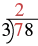Multiply the 2 in the quotient by 3 and write the product, 6, under the 7.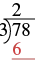Subtract that product from the first digit in the dividend. Subtract $7−67−6$. Write the difference, 1, under the first digit in the dividend.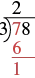Bring down the next digit of the dividend. Bring down the 8.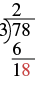Divide 18 by the divisor, 3. The divisor 3 goes into 18 six times.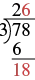Write 6 in the quotient above the 8. Multiply the 6 in the quotient by the divisor and write the product, 18, under the dividend. Subtract 18 from 18.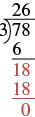We would repeat the process until there are no more digits in the dividend to bring down. In this problem, there are no more digits to bring down, so the division is finished.

$So78÷3=26.So78÷3=26.$

Check by multiplying the quotient times the divisor to get the dividend. Multiply $26×326×3$ to make sure that product equals the dividend, $78.78.$

$216×3___78✓216×3___78✓$

It does, so our answer is correct.

### How To

#### Divide whole numbers.

1. Step 1. Divide the first digit of the dividend by the divisor.
If the divisor is larger than the first digit of the dividend, divide the first two digits of the dividend by the divisor, and so on.
2. Step 2. Write the quotient above the dividend.
3. Step 3. Multiply the quotient by the divisor and write the product under the dividend.
4. Step 4. Subtract that product from the dividend.
5. Step 5. Bring down the next digit of the dividend.
6. Step 6. Repeat from Step 1 until there are no more digits in the dividend to bring down.
7. Step 7. Check by multiplying the quotient times the divisor.

### Example 1.61

Divide $2,596÷4.2,596÷4.$ Check by multiplying:

### Try It 1.121

Divide. Then check by multiplying: $2,636÷42,636÷4$

### Try It 1.122

Divide. Then check by multiplying: $2,716÷42,716÷4$

### Example 1.62

Divide $4,506÷6.4,506÷6.$ Check by multiplying:

### Try It 1.123

Divide. Then check by multiplying: $4,305÷5.4,305÷5.$

### Try It 1.124

Divide. Then check by multiplying: $3,906÷6.3,906÷6.$

### Example 1.63

Divide $7,263÷9.7,263÷9.$ Check by multiplying.

### Try It 1.125

Divide. Then check by multiplying: $4,928÷7.4,928÷7.$

### Try It 1.126

Divide. Then check by multiplying: $5,663÷7.5,663÷7.$

So far all the division problems have worked out evenly. For example, if we had $2424$ cookies and wanted to make bags of $88$ cookies, we would have $33$ bags. But what if there were $2828$ cookies and we wanted to make bags of $8?8?$ Start with the $2828$ cookies as shown in Figure 1.14.

Figure 1.14

Try to put the cookies in groups of eight as in Figure 1.15.

Figure 1.15

There are $33$ groups of eight cookies, and $44$ cookies left over. We call the $44$ cookies that are left over the remainder and show it by writing R4 next to the $3.3.$ (The R stands for remainder.)

To check this division we multiply $33$ times $88$ to get $24,24,$ and then add the remainder of $4.4.$

$3×8___24+4___283×8___24+4___28$

### Example 1.64

Divide $1,439÷4.1,439÷4.$ Check by multiplying.

### Try It 1.127

Divide. Then check by multiplying: $3,812÷8.3,812÷8.$

### Try It 1.128

Divide. Then check by multiplying: $4,319÷8.4,319÷8.$

### Example 1.65

Divide and then check by multiplying: $1,461÷13.1,461÷13.$

### Try It 1.129

Divide. Then check by multiplying: $1,493÷13.1,493÷13.$

### Try It 1.130

Divide. Then check by multiplying: $1,461÷12.1,461÷12.$

### Example 1.66

Divide and check by multiplying: $74,521÷241.74,521÷241.$

### Try It 1.131

Divide. Then check by multiplying: $78,641÷256.78,641÷256.$

### Try It 1.132

Divide. Then check by multiplying: $76,461÷248.76,461÷248.$

### Translate Word Phrases to Math Notation

Earlier in this section, we translated math notation for division into words. Now we’ll translate word phrases into math notation. Some of the words that indicate division are given in Table 1.8.

Operation Word Phrase Example Expression
Division divided by
quotient of
divided into
$1212$ divided by $44$
the quotient of $1212$ and $44$
$44$ divided into $1212$
$12÷412÷4$
$124124$
$12/412/4$
$412412$
Table 1.8

### Example 1.67

Translate and simplify: the quotient of $5151$ and $17.17.$

### Try It 1.133

Translate and simplify: the quotient of $9191$ and $13.13.$

### Try It 1.134

Translate and simplify: the quotient of $5252$ and $13.13.$

### Divide Whole Numbers in Applications

We will use the same strategy we used in previous sections to solve applications. First, we determine what we are looking for. Then we write a phrase that gives the information to find it. We then translate the phrase into math notation and simplify it to get the answer. Finally, we write a sentence to answer the question.

### Example 1.68

Cecelia bought a $160-ounce160-ounce$ box of oatmeal at the big box store. She wants to divide the $160160$ ounces of oatmeal into $8-ounce8-ounce$ servings. She will put each serving into a plastic bag so she can take one bag to work each day. How many servings will she get from the big box?

### Try It 1.135

Marcus is setting out animal crackers for snacks at the preschool. He wants to put $99$ crackers in each cup. One box of animal crackers contains $135135$ crackers. How many cups can he fill from one box of crackers?

### Try It 1.136

Andrea is making bows for the girls in her dance class to wear at the recital. Each bow takes $44$ feet of ribbon, and $3636$ feet of ribbon are on one spool. How many bows can Andrea make from one spool of ribbon?

### Section 1.5 Exercises

#### Practice Makes Perfect

Use Division Notation

In the following exercises, translate from math notation to words.

343.

$54 ÷ 9 54 ÷ 9$

344.

$56 7 56 7$

345.

$32 8 32 8$

346.

$6 42 6 42$

347.

$48 ÷ 6 48 ÷ 6$

348.

$63 9 63 9$

349.

$7 63 7 63$

350.

$72 ÷ 8 72 ÷ 8$

Model Division of Whole Numbers

In the following exercises, model the division.

351.

$15 ÷ 5 15 ÷ 5$

352.

$10 ÷ 5 10 ÷ 5$

353.

$14 7 14 7$

354.

$18 6 18 6$

355.

$4 20 4 20$

356.

$3 15 3 15$

357.

$24 ÷ 6 24 ÷ 6$

358.

$16 ÷ 4 16 ÷ 4$

Divide Whole Numbers

In the following exercises, divide. Then check by multiplying.

359.

$18 ÷ 2 18 ÷ 2$

360.

$14 ÷ 2 14 ÷ 2$

361.

$27 3 27 3$

362.

$30 3 30 3$

363.

$4 28 4 28$

364.

$4 36 4 36$

365.

$45 5 45 5$

366.

$35 5 35 5$

367.

$72 / 8 72 / 8$

368.

$8 64 8 64$

369.

$35 7 35 7$

370.

$42 ÷ 7 42 ÷ 7$

371.

$15 15 15 15$

372.

$12 12 12 12$

373.

$43 ÷ 43 43 ÷ 43$

374.

$37 ÷ 37 37 ÷ 37$

375.

$23 1 23 1$

376.

$29 1 29 1$

377.

$19 ÷ 1 19 ÷ 1$

378.

$17 ÷ 1 17 ÷ 1$

379.

$0 ÷ 4 0 ÷ 4$

380.

$0 ÷ 8 0 ÷ 8$

381.

$5 0 5 0$

382.

$9 0 9 0$

383.

$26 0 26 0$

384.

$32 0 32 0$

385.

$12 0 12 0$

386.

$16 0 16 0$

387.

$72 ÷ 3 72 ÷ 3$

388.

$57 ÷ 3 57 ÷ 3$

389.

$96 8 96 8$

390.

$78 6 78 6$

391.

$5 465 5 465$

392.

$4 528 4 528$

393.

$924 ÷ 7 924 ÷ 7$

394.

$861 ÷ 7 861 ÷ 7$

395.

$5,226 6 5,226 6$

396.

$3,776 8 3,776 8$

397.

$4 31,324 4 31,324$

398.

$5 46,855 5 46,855$

399.

$7,209 ÷ 3 7,209 ÷ 3$

400.

$4,806 ÷ 3 4,806 ÷ 3$

401.

$5,406 ÷ 6 5,406 ÷ 6$

402.

$3,208 ÷ 4 3,208 ÷ 4$

403.

$4 2,816 4 2,816$

404.

$6 3,624 6 3,624$

405.

$91,881 9 91,881 9$

406.

$83,256 8 83,256 8$

407.

$2,470 ÷ 7 2,470 ÷ 7$

408.

$3,741 ÷ 7 3,741 ÷ 7$

409.

$8 55,305 8 55,305$

410.

$9 51,492 9 51,492$

411.

$431,174 5 431,174 5$

412.

$297,277 4 297,277 4$

413.

$130,016 ÷ 3 130,016 ÷ 3$

414.

$105,609 ÷ 2 105,609 ÷ 2$

415.

$15 5,735 15 5,735$

416.

$4,933 21 4,933 21$

417.

$56,883 ÷ 67 56,883 ÷ 67$

418.

$43,725 / 75 43,725 / 75$

419.

$30,144 314 30,144 314$

420.

$26,145 ÷ 415 26,145 ÷ 415$

421.

$273 542,195 273 542,195$

422.

$816,243 ÷ 462 816,243 ÷ 462$

Mixed Practice

In the following exercises, simplify.

423.

$15 ( 204 ) 15 ( 204 )$

424.

$74 · 391 74 · 391$

425.

$256 − 184 256 − 184$

426.

$305 − 262 305 − 262$

427.

$719 + 341 719 + 341$

428.

$647 + 528 647 + 528$

429.

$25 875 25 875$

430.

$1104 ÷ 23 1104 ÷ 23$

Translate Word Phrases to Algebraic Expressions

In the following exercises, translate and simplify.

431.

the quotient of $4545$ and $1515$

432.

the quotient of $6464$ and $1616$

433.

the quotient of $288288$ and $2424$

434.

the quotient of $256256$ and $3232$

Divide Whole Numbers in Applications

In the following exercises, solve.

435.

Trail mix Ric bought $6464$ ounces of trail mix. He wants to divide it into small bags, with $22$ ounces of trail mix in each bag. How many bags can Ric fill?

436.

Crackers Evie bought a $4242$ ounce box of crackers. She wants to divide it into bags with $33$ ounces of crackers in each bag. How many bags can Evie fill?

437.

Astronomy class There are $125125$ students in an astronomy class. The professor assigns them into groups of $5.5.$ How many groups of students are there?

438.

Flower shop Melissa’s flower shop got a shipment of $152152$ roses. She wants to make bouquets of $88$ roses each. How many bouquets can Melissa make?

439.

Baking One roll of plastic wrap is $4848$ feet long. Marta uses $33$ feet of plastic wrap to wrap each cake she bakes. How many cakes can she wrap from one roll?

440.

Dental floss One package of dental floss is $5454$ feet long. Brian uses $22$ feet of dental floss every day. How many days will one package of dental floss last Brian?

Mixed Practice

In the following exercises, solve.

441.

Miles per gallon Susana’s hybrid car gets $4545$ miles per gallon. Her son’s truck gets $1717$ miles per gallon. What is the difference in miles per gallon between Susana’s car and her son’s truck?

442.

Distance Mayra lives $5353$ miles from her mother’s house and $7171$ miles from her mother-in-law’s house. How much farther is Mayra from her mother-in-law’s house than from her mother’s house?

443.

Field trip The $4545$ students in a Geology class will go on a field trip, using the college’s vans. Each van can hold $99$ students. How many vans will they need for the field trip?

444.

Potting soil Aki bought a $128128$ ounce bag of potting soil. How many $44$ ounce pots can he fill from the bag?

445.

Hiking Bill hiked $88$ miles on the first day of his backpacking trip, $1414$ miles the second day, $1111$ miles the third day, and $1717$ miles the fourth day. What is the total number of miles Bill hiked?

446.

Reading Last night Emily read $66$ pages in her Business textbook, $2626$ pages in her History text, $1515$ pages in her Psychology text, and $99$ pages in her math text. What is the total number of pages Emily read?

447.

Patients LaVonne treats $1212$ patients each day in her dental office. Last week she worked $44$ days. How many patients did she treat last week?

448.

Scouts There are $1414$ boys in Dave’s scout troop. At summer camp, each boy earned $55$ merit badges. What was the total number of merit badges earned by Dave’s scout troop at summer camp?

#### Writing Exercises

449.

Contact lenses Jenna puts in a new pair of contact lenses every $1414$ days. How many pairs of contact lenses does she need for $365365$ days?

450.

Cat food One bag of cat food feeds Lara’s cat for $2525$ days. How many bags of cat food does Lara need for $365365$ days?

#### Everyday Math

451.

Explain how you use the multiplication facts to help with division.

452.

Oswaldo divided $300300$ by $88$ and said his answer was $3737$ with a remainder of $4.4.$ How can you check to make sure he is correct?

#### Self Check

After completing the exercises, use this checklist to evaluate your mastery of the objectives of this section.

Overall, after looking at the checklist, do you think you are well-prepared for the next Chapter? Why or why not?

Order a print copy

As an Amazon Associate we earn from qualifying purchases.